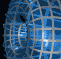Português | English Beyond 3D > Torus Triptych > Mathematics

# The Mathematics of Torus Triptych

A torus can be generated by rotating a circle around an axis in the same plane as the circle, but not intersecting it. We can produce parametric equations for such a torus of revolution as follows: if we consider a circle of radius b in the xz-plane, centered at the point (x, z) = (a, 0) on the x-axis, then the points on the circle are given by (x, z) = (a + b cos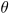, b sin). If we rotate this circle about the z-axis, then each point (x, z) on the original circle traces out a new circle in a plane parallel to the xy-plane; the radius of this new circle will be x (the distance of the original point from the z-axis), and the height of the plane containing the new circle will be z. This means the new circle can be parameterized by (x cos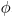, x sin, z). As we let (x, y) vary over the entire original circle, we obtain a parameterization for the torus:

T(,) = ((a + b cos) cos, (a + b cos) sin, b sin).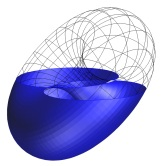In "Torus Triptych" we used a =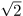and b = 1. This basic torus was rotated to three different positions and then sliced by a horizontal plane at various heights to obtain the three sequences presented. The lower sequence (in blue) has a particularly interesting slice in the second image. Here, the horizontal plane intersects the torus in two overlapping circles of equal radius.